#### Get Our New Book!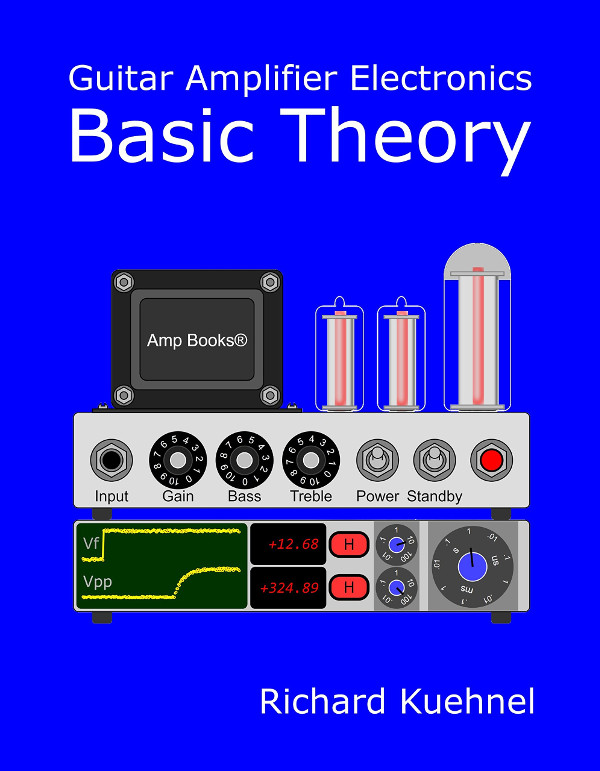In working with complex impedances you frequently find yourself writing j times 2 times pi times f, over and over again. Some people like to shorten this by using radians. This leaves you with only writing j times omega. Personally, I like to carry this efficiency to the ultimate extreme by borrowing from Pierre-Simon Laplace. I use one letter to represent all four terms: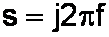The Laplace transform is used by engineers to solve linear ordinary differential equations. It essentially transforms a complicated calculus problem into a simple algebra problem. Fortunately we don't need to know anything about the calculus that the Laplace transform represents. Instead, let's just stick with the algebra.

#### Laplace for Inductors

Under certain mathematical conditions which have no relevancy to the treble response of your Fender Deluxe 5E3, the impedance of an inductor, expressed in terms of Laplace, is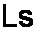When we substitute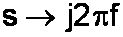We recognize the impedance of an inductor as a function of frequency and inductance: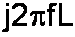Our Laplace version is certainly shorter, but we could have chosen any letter. By sticking with s we are consistent with current usage. Show an electronic engineer the term Ls and you'll get instant recognition. Try to use Lt or Lu and you'll have some serious explaining to do. In that case don't refer your EE friends to me - you're on your own!

#### Laplace for Capacitors

According to Laplace, a capacitor's impedance is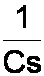This time when we substitutewe get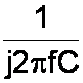This doesn't quite look right, because j is in the denominator instead of the numerator. To get a more familiar representation, we multiply the numerator and denominator by j: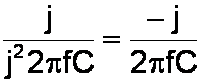Did I really say "multiply by j?" The sound you hear is Laplace rolling over in his grave! As I said in the tutorial on imaginary numbers, j is a symbol indicating that a number is imaginary. It is not actually a number itself. But to save some extra steps and some extra mathematical jargon, this technique works for me. As Munro Saki once said, "a little approximation sometimes saves tons of explanation."

#### Laplace for Resistors

The Laplace transform representation of a resistor with a value of R ohms is simply, drumroll please... , R. Now that was easy!

#### Plotting the Frequency Response

To plot a frequency response expressed in terms of the Laplace transform, we first substituteand then take the magnitude of the result. We'll see an example in the next problem

#### Problem

Consider again the Fender Harvard 5F10 input circuit. The resistance R and the Miller capacitance C form a voltage divider. What is the magnitude of the output voltage compared to the input voltage as a function of frequency?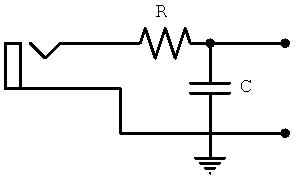#### Solution

The resistor and the Miller capacitance combine to form a voltage divider, where Z1 = R and Z2 = 1/Cs. The output voltage isThe frequency response is thusThis is an extremely compact form, which is why engineers like me love it. We don't get out much. This equation is also a complete description, because it contains both magnitude and phase information, expressed by using complex values. To plot the magnitude of the frequency response, we first substituteto getWe multiply the numerator and the denominator by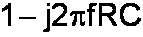to eliminate the imaginary term in the denominator:which simplifies to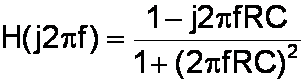Next we separate the real and the imaginary terms: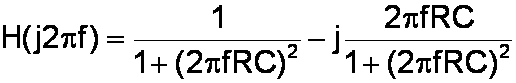To convert the complex response to a magnitude response, we square the real and imaginary components, add them together, and take the square root of the total.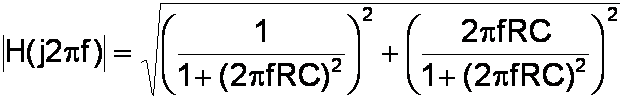This gives us the magnitude of the frequency response:That's it! We've been using the Pierre-Simon Laplace Transform, an advanced mathematical concept that lives in the halls of Princeton, MIT, and Technical University Berlin.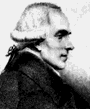The nice thing is, we don't need to know how to design a Boeing 737 to fly one to Cincinnati.Guitar Amplifier Electronics: Basic Theory - a modern approach to understanding tube amp design. Learn more.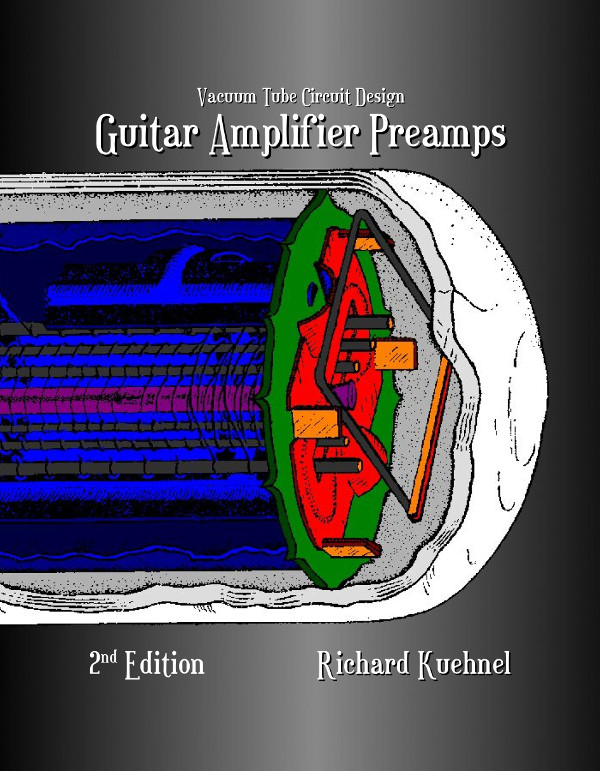Guitar Amplifier Preamps - upstream circuits using triodes and pentodes. Learn more.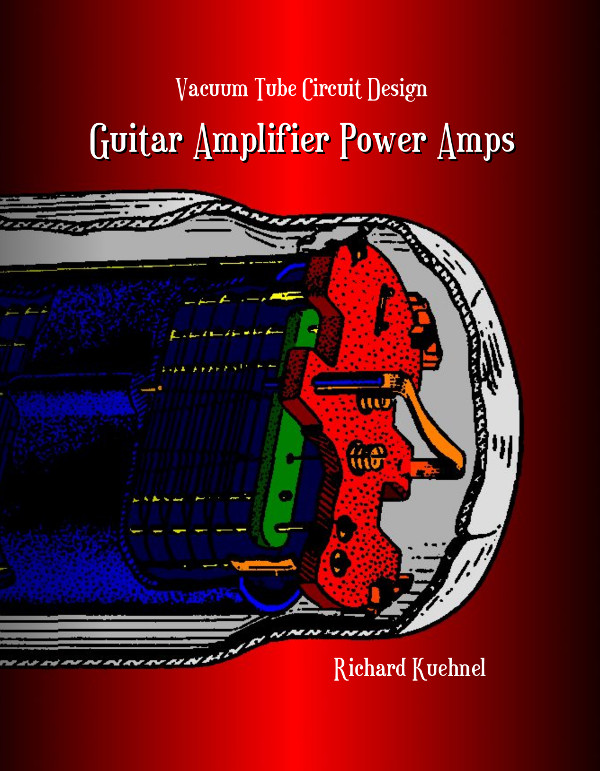Guitar Amplifier Power Amps - from the phase inverter to the loudspeaker. Learn more.The Fender Bassman 5F6-A - plus Marshall's Bluesbreaker and Plexi. Learn more.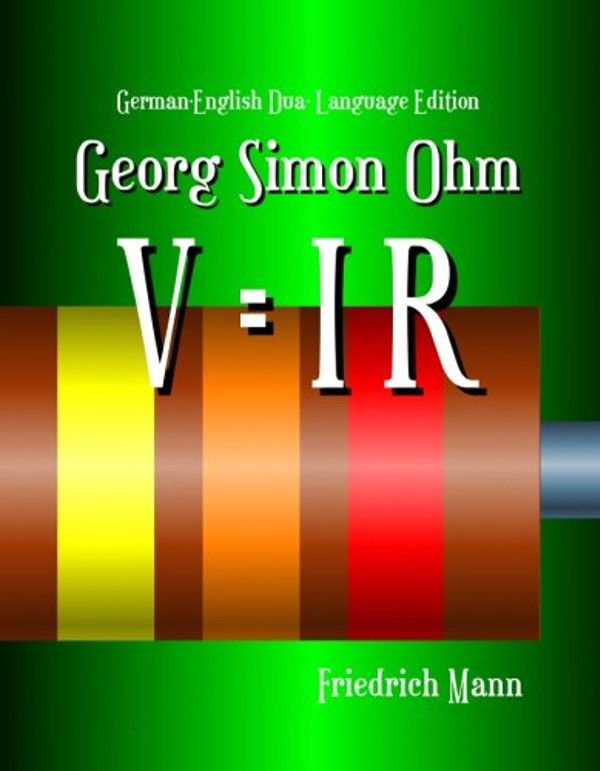Georg Simon Ohm - Imagine a world without Ohm's Law. Learn more.